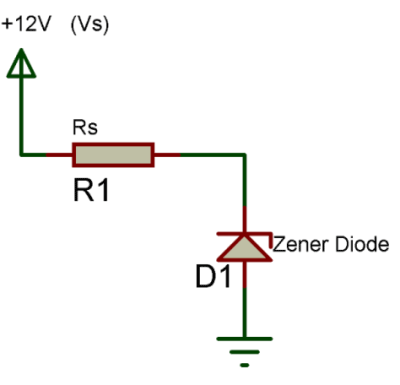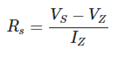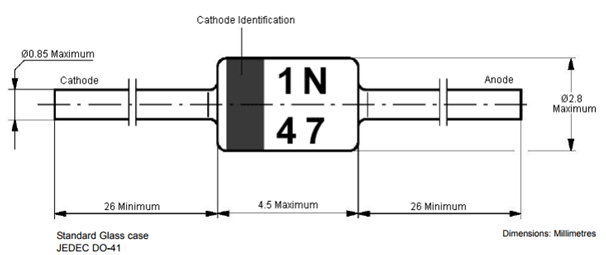# 3.9V Zener Diode - 1N4730A

[Click the image to enlarge it]

### Pin Configuration

 Pin No. Pin Name Description 1 Anode Current always Enters through Anode 2 Cathode Current always Exits through Cathode

### Features

• Nominal Zener Voltage (VZ): 3.9V
• Power dissipation (PZ): 1300mW (practically 500mW)
• Zener regulator current (IZm): 234mA
• Package: DO-41

Note: Complete Technical Details can be found at the 1N4730A datasheet given at the end of this page.

### 1N4730A Equivalent Zener Diodes

4.7V Zener, 5.1V Zener, 6.8V Zener, 7.5V Zener, 15V Zener

### How to select a Zener Diode

A Zener diode is another form of diode, but is used for entirely different purpose. They are mainly used in protection circuits or as crude voltage regulators. Let us see how we can select one for your application.

There are two main parameters that are to be checked while selecting a Zener diode. One is the Zener Voltage and the other is Power dissipation. The Zener voltage is the voltage which appears across the Zener diode when a higher reverse potential voltage is applied to it. In regulator circuits it is this voltage (Zener voltage) which will be regulated and in protection circuit it is this voltage (Zener voltage) more than which the circuit is protected. The power dissipation decides the amount of current that can flow through the diode. Higher the power dissipation higher the current can flow.

### How to use a Zener Diode

As said a Zener diode will mostly be used in a protection circuit or in a crude voltage regulator circuit. Either way, it very important to remember that a Zener diode should always be used along with a Zener resistor.

A Zener resistor is nothing but an ordinary resistor which is used for current limiting purpose. This resistor decides (limits) the amount of current that can flow through the Zener diode or through the load connected to Zener diode; this was the Zener diode is protected from high current. If this resistor is not used the diode will be damaged due to high current. A simple Zener diode circuit is shown below.In the above circuit the formulae to calculate the Zener series resistor Rs is shown belowFor a 1N4730A Zener diode the value of Vz is 3.9V and Pz is 500mW as mentioned in specifications above, now with supply voltage (Vs) of 12V the value of Rs will be

Rs = (12-3.9)/Iz

Iz = Pz/Vz = 500mW / 3.9V = ~123mA

Therefore, Rs = (12-3.9)/123 = 0.0576 = 65 ohms

Rs = 65ohms (approx)

### Applications of Diode

• Used in Voltage protection circuits
• Can be used as low current voltage regulator
• Input voltage protection for Microcontrollers or other IC’s
• Voltage stabilizing circuits

### 2D representation (DO-41)Component Datasheet